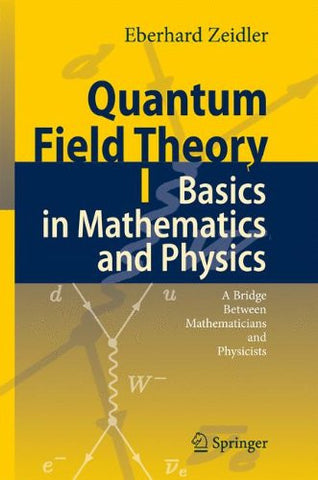# Quantum Field Theory I: Basics in Mathematics and Physics: A Bridge between Mathematicians and Physicists (v. 1)

• \$279.88
• Save \$-25.43
• Binding: Hardcover
• Author: Eberhard Zeidler
• Publish Date: 2011-04-06

Attention : For textbook, access codes and supplements are not guaranteed with used items.

This is the first volume of a modern introduction to quantum field theory which addresses both mathematicians and physicists, at levels ranging from advanced undergraduate students to professional scientists. The book bridges the acknowledged gap between the different languages used by mathematicians and physicists. For students of mathematics the author shows that detailed knowledge of the physical background helps to motivate the mathematical subjects and to discover interesting interrelationships between quite different mathematical topics. For students of physics, fairly advanced mathematics is presented, which goes beyond the usual curriculum in physics.